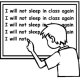Home > Grade 8 > Square Root Expression

# Square Root Expression

Directions: Use the digits 1 to 9, at most one time each, to fill in the boxes to make the following expression as close to 0 as possible.### Hint

What are all the perfect square three digit numbers? Several of these can’t be used since they contain a repeated digit (e.g. 121 uses the digit 1 twice and 144 uses the digit 4 twice)

### Answer

Using Perfect squares:
Sqrt(256) – sqrt(81) – sqrt(9) = 4

Using non-perfect squares:
Sqrt(145) – sqrt(92) – sqrt(6) = 0.00044178938368

Source: Erick Lee

## Minimize Slope

Directions: Given the point (3,5), use digits 1-9, at most one time, to find a …

### 4 comments

1.Dang it.

I got:
√124 – √89 – √3 = -0.030503214

•You tried. That is what is important!

2.Should it not be √256 – √81 -√49 = 0 ?

•Your answer looks correct. Maybe the wording needs to be clarified? It says as close to zero, maybe the question needs to state without equaling zero?